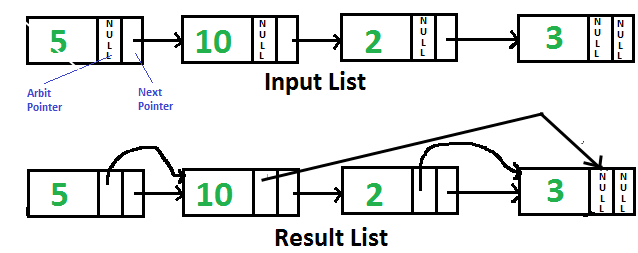# Python Program For Pointing Arbit Pointer To Greatest Value Right Side Node In A Linked List

• Last Updated : 18 May, 2022

Given singly linked list with every node having an additional “arbitrary” pointer that currently points to NULL. We need to make the “arbitrary” pointer to the greatest value node in a linked list on its right side.A Simple Solution is to traverse all nodes one by one. For every node, find the node which has the greatest value on the right side and change the next pointer. The Time Complexity of this solution is O(n2).

An Efficient Solution can work in O(n) time. Below are the steps.

1. Reverse the given linked list.
2. Start traversing the linked list and store the maximum value node encountered so far. Make arbit of every node to point to max. If the data in the current node is more than the max node so far, update max.

Following is the implementation of the above steps.

## Python

 `# Python Program to point arbit pointers ``# to highest value on its right`` ` `# Node class ``class` `Node: `` ` `    ``# Constructor to initialize the ``    ``# node object ``    ``def` `__init__(``self``, data): ``        ``self``.data ``=` `data ``        ``self``.``next` `=` `None``        ``self``.arbit ``=` `None`` ` `# Function to reverse the linked list ``def` `reverse(head):``    ``prev ``=` `None``    ``current ``=` `head``    ``next` `=` `None`` ` `    ``while` `(current !``=` `None``):    ``        ``next` `=` `current.``next``        ``current.``next` `=` `prev``        ``prev ``=` `current``        ``current ``=` `next``     ` `    ``return` `prev`` ` `# This function populates arbit pointer ``# in every node to the greatest value ``# to its right.``def` `populateArbit(head):`` ` `    ``# Reverse given linked list``    ``head ``=` `reverse(head)`` ` `    ``# Initialize pointer to maximum ``    ``# value node``    ``max` `=` `head`` ` `    ``# Traverse the reversed list``    ``temp ``=` `head.``next``    ``while` `(temp !``=` `None``):``     ` `        ``# Connect max through arbit``        ``# pointer``        ``temp.arbit ``=` `max`` ` `        ``# Update max if required``        ``if` `(``max``.data < temp.data):``            ``max` `=` `temp`` ` `        ``# Move ahead in reversed list``        ``temp ``=` `temp.``next``     ` `    ``# Reverse modified linked list and ``    ``# return head.``    ``return` `reverse(head)`` ` `# Utility function to print result ``# linked list``def` `printNextArbitPointers(node):`` ` `    ``print``(``"Node    "``, ``          ``"Next Pointer    "` `, ``          ``"Arbit Pointer"``)``    ``while` `(node !``=` `None``):``     ` `        ``print``(node.data , ``              ``"        "``, ``              ``end ``=` `"")`` ` `        ``if` `(node.``next` `!``=` `None``):``            ``print``(node.``next``.data , ``                  ``"        "``, end ``=` `"")``        ``else` `:``            ``print``(``"None"` `, ``                  ``"        "``,end ``=` `"")`` ` `        ``if` `(node.arbit !``=` `None``):``            ``print``(node.arbit.data, end ``=` `"")``        ``else` `:``            ``print``(``"None"``, end ``=` `"")``         ` `        ``print``("")``        ``node ``=` `node.``next``     ` `# Function to create a new node ``# with given data ``def` `newNode(data):`` ` `    ``new_node ``=` `Node(``0``)``    ``new_node.data ``=` `data``    ``new_node.``next` `=` `None``    ``return` `new_node`` ` `# Driver code``head ``=` `newNode(``5``)``head.``next` `=` `newNode(``10``)``head.``next``.``next` `=` `newNode(``2``)``head.``next``.``next``.``next` `=` `newNode(``3``)``head ``=` `populateArbit(head)`` ` `print``(``"Resultant Linked List is: "``)``printNextArbitPointers(head)``# This code is contributed by Arnab Kundu`

Output:

```Resultant Linked List is:
Node    Next Pointer    Arbit Pointer
5               10              10
10              2               3
2               3               3
3               NULL            NULL```

Recursive Solution:
We can recursively reach the last node and traverse the linked list from the end. The recursive solution doesn’t require reversing of the linked list. We can also use a stack in place of recursion to temporarily hold nodes. Thanks to Santosh Kumar Mishra for providing this solution.

## Python3

 `# Python3 program to point arbit pointers``# to highest value on its right `` ` `''' Link list node '''``# Node class ``class` `newNode: `` ` `    ``# Constructor to initialize the ``    ``# node object ``    ``def` `__init__(``self``, data): ``        ``self``.data ``=` `data ``        ``self``.``next` `=` `None``        ``self``.arbit ``=` `None`` ` `# This function populates arbit pointer ``# in every node to the greatest value ``# to its right. ``maxNode ``=` `newNode(``None``)``def` `populateArbit(head):``     ` `    ``# using static maxNode to keep track ``    ``# of maximum orbit node address on ``    ``# right side ``    ``global` `maxNode``     ` `    ``# if head is null simply return the list ``    ``if` `(head ``=``=` `None``):``        ``return``         ` `    ``''' if head.next is null it means we ``        ``reached at the last node just update ``        ``the max and maxNode '''``    ``if` `(head.``next` `=``=` `None``):``        ``maxNode ``=` `head ``        ``return``         ` `    ``''' Calling the populateArbit to the``        ``next node '''``    ``populateArbit(head.``next``) ``     ` `    ``''' updating the arbit node of the ``        ``current node with the maximum ``        ``value on the right side '''``    ``head.arbit ``=` `maxNode ``     ` `    ``''' if current Node value id greater ``        ``then the previous right node then ``        ``update it '''``    ``if` `(head.data > maxNode.data ``and` `        ``maxNode.data !``=` `None` `):``        ``maxNode ``=` `head ``    ``return`` ` `# Utility function to prresult ``# linked list ``def` `printNextArbitPointers(node):``    ``print``(``"Node    "` `, ``          ``"Next Pointer    "` `, ``          ``"Arbit Pointer"``)``    ``while` `(node !``=` `None``):``        ``print``(node.data, ``              ``"        "``, ``              ``end ``=` `"")``        ``if``(node.``next``):``            ``print``(node.``next``.data,``                  ``"        "``, ``                  ``end ``=` `"")``        ``else``:``            ``print``(``"NULL"``, ``"        "``, ``                   ``end ``=` `"")``        ``if``(node.arbit):``            ``print``(node.arbit.data, end ``=` `"")``        ``else``:``            ``print``(``"NULL"``, end ``=` `"")``        ``print``()``        ``node ``=` `node.``next``         ` `# Driver code``head ``=` `newNode(``5``) ``head.``next` `=` `newNode(``10``) ``head.``next``.``next` `=` `newNode(``2``) ``head.``next``.``next``.``next` `=` `newNode(``3``) ``populateArbit(head) ``print``(``"Resultant Linked List is:"``) ``printNextArbitPointers(head) ``# This code is contributed by SHUBHAMSINGH10`

Output:

```Resultant Linked List is:
Node    Next Pointer    Arbit Pointer
5               10              10
10              2               3
2               3               3
3               NULL            NULL```

Please refer complete article on Point arbit pointer to greatest value right side node in a linked list for more details!

My Personal Notes arrow_drop_up